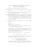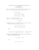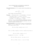# Lecture notes pdfLoading previews...probability - addition, multiplication, independence, Bayes' formularandom variables, cdf, and transformationsexpected value, moments, variance, standard deviationBernoulli trials, Binomial distribution - cdf and use of tablesderivation of the poisson distribution and its properties
13 files in this resource

## Lecture notes pdf

Lecture notes in PDFView Item